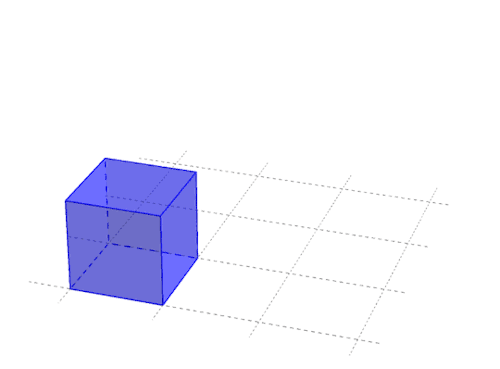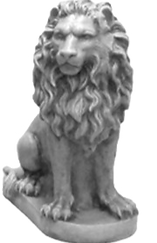top of page#### the Cube

The principal form within Quantum Geometry is the Cube. It determines the layout of the SpaceTime Fabric at quantum level and all of the other geometric forms that create at this level are born out of the Cube. In Alchemy this six sided geometry is known as Metatron's Cube. Even when the cube is drawn in 2D perspective (which we always need to do when working in all of the 2D media) you see immediately a 3D form. It is the most basic 3D geometry that we can draw and is seen as the representative of the physical world, of matter itself.

When working on deciphering the Crop Circle Code for this amazing pattern from 2007 at West Overton this wonderful cube pattern appeared. This was a magical moment when so many of the puzzle pieces fell into place. Our Universe is geometric, at all levels, and the Cube sets the basic 3D pattern for this geometry. You also see directly that the 2D square becomes a diamond (rhomboid) when creating a cube in 3D perspective, this form links Heaven and Earth in Alchemy and is the symbol for the Christ, it is the foem we can use to decipher the geometry of the Universe.

If you look at the following three forms, they are all directly associated with the Cube, they are all born out of the cube. In the middle is the octahedron, on the left the cube-octahedron, and on the right the star-tetrahedron. Each of these forms is made using only equilateral triangles but put together in a way that they form these 3D forms. The star-tetrahedron, with 8 points fits perfectly into a cube, with each point touching the 8 internal corners of the cube. The octahedron fits perfectly into the cube with each of its 6 points touching the middle point of the 6 sides of the cube. And the cube-octahedron is a cube with each of its 8 corners removed forming an equilateral triangle shaped surface where the corner was.At the level of Quantum Geometry these three forms create the basic geometry of the four fundamental particles that make up the atom. They are the building blocks of all of matter, everywhere, whether it is an oxygen atom in a water droplet on a sunflower petal, or a raging fire of hydrogen atoms at the surface of a star. You can read more about these three forms on the page about the Atom.The cube is busy everywhere with its own form of geometrical alchemy. Here yo can see the diamond form as the base of the cube in 2D perspective, joining the single cube, in the top left of the drawing, with two cubes, four cubes and then eight cubes. Then it turns into one whole cube again, at the next level. This fractal operation could go on infinitely, and maybe does, forming the universal matrix of matter.The cube is the base geometry of the SpaceTime fabric, the quantum world, and all of the universe. This has given the cube mystical powers and it has been seen as a universal generator of endless energy. Making it a very desired object, not just for seekers for a new energy that could be used to break us free of our slavery to fossil fuels, but also for power seekers who would use for less moral reasons.

In its higher energy form the cube is seen to float in space as a higher dimensional object known as the Tesseract or hypercube. The geometry of the cube when moved through into the fourth dimension creates the double cube, or cube within a cube, that can be seen in the drawing of Metatron's Cube. It is also seen as a transformation portal from the fourth to the fifth dimension . . taking you through the Lions Gate to the New Jerusalem.bottom of page